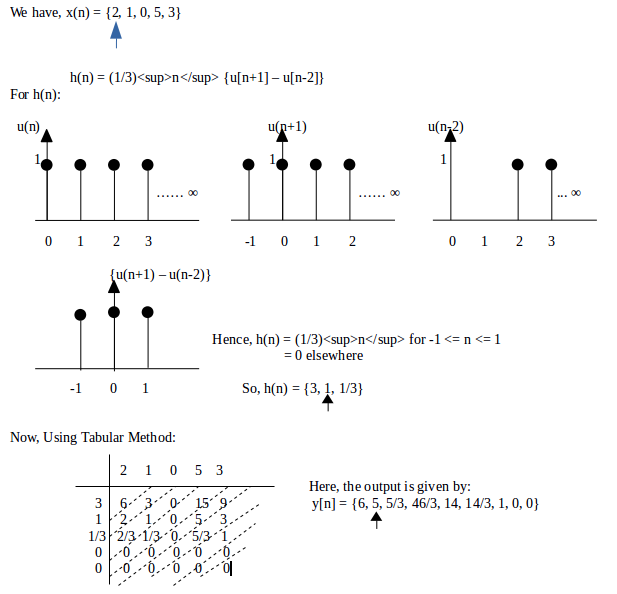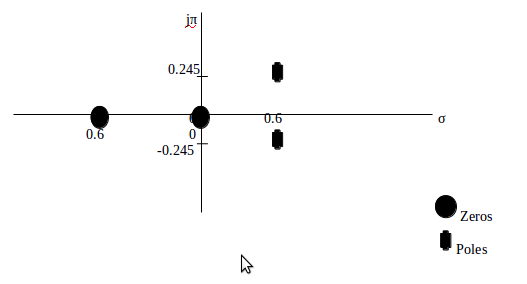### Notes of Digital Signal Analysis and Processing [CT 704]

#### Analysis of LTI system in Frequency Domain

Frequency Response of LTI System; Response to Complex Exponential

LTI System

- Any system which follows both linearity and time invariance is called LTI system.

- To be linear;
If x1[n] ------→ y1[n] and x2[n] -----→ y2[n]
then,
x[n] = Ax1[n] + Bx2[n] --→ y[n] = Ay1[n] + By2[n]

- To be time invariant:
If x[n] ---→ y[n]
then,
x[n-no] -----→ y[n-no]

Impulse Response

- Impulse response is the output of a LTI system when the input is an unit impulse response.
- It is represented as h[n].
- For any LTI system with input x[n] and impulse response h[n], the output signal y[n] is given by:
y[n] = ∑k = -∞ to ∞ x[k] h[n-k] = x[n] * h[n]
- This output is known as convolution sum.

Convolution Sum

1. Graphical Method:
The steps involved are as follows:
a) Fold h(k) about k = 0 to obtain h(-k)
b) Shift h(-k) by no to right (if no is positive) or to left (no is negative) to obtain h(no - k)
c) Multiply x(k) by h(no - k) to obtain product sequence.
d) Sum all values of product sequence to obtain value of output at time n = no.

2. Tabular Method:
The steps involved are as follows:
a) Form a table with horizontal header as the elements of x(n) and vertical header as the elements of h(n).
b) Multiply x(n) and h(n) for each possible combinations and write in the corresponding table cell.
c) Partition the cells diagonally right to left from left side of the table.
d) The corresponding y is obtained by sum of elements present in the partition.

Q) Obtain output if x(n) = {2, 1, 0, 5, 3} and h(n) = (1/3)<sup>n</sup> {u[n+1] – u[n-2]}.

Solution:Properties of Convolution Sum:

1. Commutative
x[n] * h[n] = h[n] * x[n]

2. Distributive
x[n] * {h1[n] + h2[n]} = {x[n] * h1[n]} + {x[n] * h2[n]}

3. Associative
x[n] * {h1[n] * h2[n]} = {x[n] * h1[n]} * h2[n]

Stability:

- A LTI system is stable if its impulse response is absolutely summable.
∑k = -∞ to ∞ |h(k)| <= M < ∞

Causality:

- A LTI system is causal if its impulse response is causal.
h[n] = 0 for all n < 0

Memory:

- A LTI system is memory less if:
h[n] = 0 for all n != 0

Linear Constant Coefficient Difference Equation and Corresponding System Function

- A general Nth order linear constant coefficient difference equation is stated in the form:

```      y(n) = -∑k=1toN {ak y(n-k)} + ∑k=0toM {bk x(n-k)}
where, ak and bk are constant coefficients
N is the order of difference equation.
```

- The corresponding system function or transfer function is given by:

```       H(z) = Y(z) / X(z) = [ ∑k=0toM {bk z-k} ] / [ ∑k=0toN {ak z-k} ]
```

- The difference equation specifies a digital filtering operations and the coefficients fully characterize the filter.

- The order of difference equation corresponds to the memory of the system being represented.

Relationship of Frequency Response to Pole-Zero System

Q) Determine output of the sequence with h[n] = (1/2)<sup>n</sup> u[n] when the input signal is x[n] = 10 – 5sin(πn/2) + 20cos(πn) for -∞ < n < ∞.

```Given:
x[n] = 10 – 5sin(πn/2) + 20cos(πn) for -∞ < n < ∞
h[n] = (1/2)n u[n]```

Taking Discrete Time Fourier Transform of h[n]:
H(w) = ∑n=-∞to∞ {h[n] e-jwn}
= ∑n=0to∞ {(1/2)n e-jwn}
= 1 / {1- ½ . e-jw}
= 1 / {1 – ½ (cos w – j sin w)}

At w= 0 ; H(0) = 2
At w = π/2 ; H(π/2) = 2 / (2 + j)
At w = π ; H(π) = 2 / 3

Now,
The output of the system is :
y[n] = H(w) . x[n]
= H(0) . 10 – H(π/2) . 5sin(πn/2) + H(π) . 20cos(πn)
= 20 – 10/√5 sin(πn/2 – 26.6) + 40/3 cos(πn) for -∞ < n < ∞

Linear Phase of LTI System and its Relationship to Causality

Q) Plot pole-zero in Z-plane and draw magnitude response of difference equation: y[n] – 0.4 y[n-1] + 0.1 y[n-2] = x[n] + 0.6 x[n-1]

```The given difference equation is:
y[n] – 0.4 y[n-1] + 0.1 y[n-2] = x[n] + 0.6 x[n-1]```

Taking z-transform on both sides;
Y(z) – 0.4 z-1 Y(z) + 0.1 z-2 Y(z) = X(z) + 0.6 z-1 X(z)
Y(z) / X(z) = {1 + 0.6 z-1} / {1 – 0.4 z-1 + 0.1 z-2}

So,
H(z) = Y(z) / X(z) = {z2 + 0.6 z} / {z2 - 0.4 z + 0.1}

H(z) = {z ( z + 0.6 ) } / {z2 - 0.4 z + 0.1}

To find zeros:
Y(z) = 0
z (z+0.6) = 0
So, z = 0 or z = -0.6

To find poles:
X(z) = 0
{z2 - 0.4 z + 0.1} = 0
So,
z = 1/5 + √6 / 10 i or z = 1/5 - √6 / 10 i

The pole – zero plot in z-plane is shown in figure below:For Magnitude Response

`     H(z) = {1 + 0.6 z-1} / {1 – 0.4 z-1 + 0.1 z-2}`

Putting z = ejw
H(ejw) = {(1+0.6cosw) – j sinw} / {(1-0.4cosw+0.1cos2w) – j (0.1sin2w+0.4sinw)}